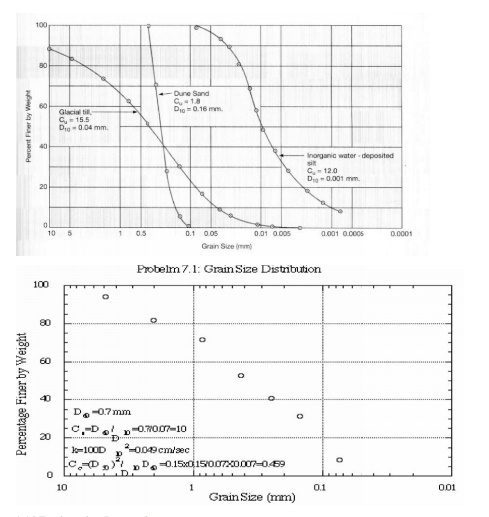Home | | Soil Mechanics | Soil Mechanics: Index properties

# Soil Mechanics: Index propertiesFirst of all, let's discuss the sieve that is the essential tool to study particle size distribution.

Soil Mechanics: Index properties

Index properties

1 Particle Size Distri bution

First of all, let's discuss the sieve that is the essential tool to study particle size distribution.

U.S. Standard Sieve Sizes

Sieve Sieve opening (mm)

4          4.75

10       2.00

20       0.850

40       0.425

60       0.250

100     0.150

200     0.074

Gradation is a measure of the distribution of a particular soil sample. Larger gradation means a wider particle size distribution. Well graded � poorly sorted (e.g., glacial till) Poorly graded � well sorted (e.g., beach sand)

The range of grain size distribution is enormous for natural soils. E.g., boulder can be ~1 m in diameter, and the colloidal mineral can be as small as 0.00001 mm = 0.01 micron. It has a tremendous range of 8 orders of magnitude.

Example: If you have a soil sample with a weight of 150 g, after thorough sieving you get the following result.

sieve   size(mm)       W(g)   %         accum%        100accum%

4          4.750 30.0   20       20       80

20       0.850 40.0   26.7   46.7   53.3

60       0.250 50.0   33.3   79       21

100     0.150 20.0   13.3   92       8

200     0.074 10.0   6.67   98       2The last column shows the percentage of material finer than that particular sieve size by weight.

There are a number of way s to characterize the particle size distribution of a particular soil sample.D10:

D10 represents a grain diameter for which 10% of the sample will be finer than it. Using another word, 10% of the sample by weight is smaller than diameter D10. It is also called the effective size and can b e used to estimate the permeability.

Hazen's approximation (a n empirical relation between hydraulic conductivity with grain size) k (cm/sec) = 100D10D 10

Where D10 is in centimeters.

It is empirical because it is not consistent in dimension (cm/sec vs cm2).

Uniformity coefficient Cu :

Cu = D60/D10

Where D60 is the diameter for which 60% of the sample is finer than D6 0.

The ratio of two characteri stic sizes is the uniformity coefficient Cu. A pparently, larger Cu means the size distribution is wider and vice versa. Cu = 1 means unif orm, all grains are in the same size, such as the case of dune sands. On the other extreme is the glacial till, for which its Cu can reach 30.

from Cu = D60/D10 , then D60 = CuD10

Coefficient of Curvature  Cc

Another shape parameter, as the second moment of grain size distribution curve, is called the coefficient of curvature, an d defined as

Cc = (D30 D30)/ (D10 D 60)

A soil is thought to be well graded if the coefficient of curvature Cc between 1 and 3,with Cu greater than 4 for gravels and 6 for sands.Study Material, Lecturing Notes, Assignment, Reference, Wiki description explanation, brief detail

Related Topics